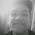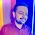## Wednesday, January 25, 2012

It is a simple project just to provide a Hang Man game concept.In this project I haven’t draw a man for a wrong choice so,try to draw a simple man by using “|” pattern in your project which make your project better .
Here is the source code ,copy and compile it in Code::blocks gcc compiler or Download project from GitHub.

`#include <iostream>`
`#include <cstdlib>`
`#include <cstdio>`
`#include <fstream>`
`#include <cstring>`
` `
`using namespace std;`
` `
`inline void type_of_word(char f);`
` `
` `
`int main()`
`{   char c,h,ch,ch1,ch2;`
`    char word;`
`    char word2;`
` `
`    int l,i,ng,n,k,x;`
` `
`do{`
`    do{`
`        c='\0';`
`        cout<<"\n\t\t    *********** HANGMAN IN C++ ***********\n\n";`
` `
`        cout<<"(E) Enter a word\n\n(C) Computer chooses word\n\n(A)" `
`        cout<<"Add new word to list\n\n(Q) Quit\n\n\nEnter your choice (E - C - Q): ";`
`        cin>>ch2;`
`       }while (ch2!='C' && ch2!='c' && ch2!='E' && ch2!= 'e' &&`
`                ch2!='Q' && ch2!= 'q'&& ch2!='A' && ch2!= 'a');`
`    if (ch2 == 'Q' || ch2=='q')  exit (0);`
` `
`    if (ch2 == 'C' || ch2=='c')`
` `
`    {`
`        ifstream fin("hangword.txt");`
`        if(!fin) {`
`        cout<<"File missing, aborting.\n\nYou are missing a file of name" `
`        cout<<"**hangword.txt**\n\nLocate it, then place it next to the" `
`        "program file.\n\n"; system("pause"); return 0;}`
`        for (i=0;!fin.eof();i++)   fin.getline(word,25);`
`        fin.close();`
` `
`        do {`
`        x=rand();`
`        }while(x>i || x<0);`
` `
`        ifstream finn("hangword.txt");`
`        for (i=0;!finn.eof();i++)`
`        {finn>>c; finn.getline(word,25); if (x==i) break;}`
`        finn.close();`
`    }`
` `
`  if (ch2 == 'A' || ch2=='a')`
` `
`    {`
`        ofstream fout("hangword.txt",ios::app);`
`        if(!fout) {//clrscr();`
`        cout<<"File missing, aborting.\n\nYou are missing a file of name"`
`        " **hangword.txt**\n\nLocate it, then place it next to the program"`
`        " file.\n\n"; system("pause"); return 0;}`
`        cin.get();`
`        cout<<"Choose the topic of your word\n\n(M) Movie\n\n(A) Animal\n\n(P)"`
`        " Sport\n\n(S) Song\n\nEnter your choice (A-P-S-M) : ";`
`        cin>>h;`
`        cin.get();`
`        //clrscr();`
`        cout<<"\n\nThe word should not exceed 25 letters\n\nEnter the word : ";`
`        cin.getline(word,25);`
`        fout<<h<<word<<endl;`
`        fout.close();`
` `
`    }`
` `
` `
`   if (ch2 == 'E' || ch2=='e')`
`     {// clrscr();`
`       cin.get();`
`       cout<<"\t\t\t Type the word :  ";`
`       cin.getline (word, 25);`
`     }`
` if (ch2 == 'E' || ch2=='e' || ch2 == 'C' || ch2=='c')`
`{`
`l=strlen(word);`
`char choosen="\0";`
`n=0;k=0;`
` `
` for(i=0;i<=24;i++)`
`   {`
`    if (word[i]=='\0') {word2[i]='\0';break;}`
`    if (word[i]==' ')  {word2[i]=' ';  n++;}`
`    if (word[i]!=' ')  word2[i]='-';`
`   }`
`ng=l+2-n;     //only 2 guesses extra`
`   do{`
`   there:  type_of_word(c);`
`     if (k!=0)  cout<<"\n\n\t\t\tChoosen letters : "<<choosen<<"\n";`
`     cout<<"\n\n\n\t\t\t      "<<word2<<"\n\n\nYou have "<<ng`
`     << " guesses left, choose a letter : ";`
`     cin>>ch; cin.get();`
`     for (i=0;i<25;i++) if (choosen[i]==ch) {//clrscr();`
`     cout<<"\a\t\t     !!You have choosen "<<ch<<" already!!\n";goto there;}`
`     ng--; choosen [k]=ch; choosen [k+1]=',';k+=2;`
` `
`     for (i=0;i<=24;i++)    `
`     if (word[i]==ch || word[i]==ch+32 || word[i]==ch-32) word2[i]=ch;`
`     if (!strcmpi (word2,word)) {cout<<"\n\t\t\t      "<<strupr(word)`
`     <<"\n\n\t\t\tCongratulations  :-()\n"; break;}`
` `
`    }while(ng>0 || !strcmpi (word2,word));`
` `
` `
`if (strcmpi (word2,word))  cout<<"\nSorry, maybe next time.\n\nThe word was : "`
`<<strupr(word)<<endl;`
`}`
` `
`cout<<"\nWould you like to play again??? (Y - N) : ";`
`cin>>ch1;  cin.get();`
` `
`}while (ch1=='y' || ch1=='Y');`
`      system("PAUSE");`
`      return 0;`
`}`
` `
`inline void type_of_word(char f)`
` `
`{    if (f=='m') cout<<"\t\t\t\tMOVIE";`
`     if (f=='a') cout<<"\t\t\t\tANIMAL";`
`     if (f=='p') cout<<"\t\t\t\tSPORT";`
`     if (f=='s') cout<<"\t\t\t\tSONG";`
`}`
` `
`// End of Code`

1.Hey why does the topic of the word doesnt appear in the program? only the sport topic shows

1.i dont understand this program after i've run it.can u explain for me?

2.This comment has been removed by the author.

3.what are purpose of hangman game plz give me ans

4.what are purpose of hangman game plz give me ans

2.but it was not working

3.There is one missing semicolon...

cout<<"(E) Enter a word\n\n(C) Computer chooses word\n\n(A)";

and a broken wrapped line, replace with...
cout<<"File missing, aborting.\n\nYou are missing a file of name **hangword.txt**\n\nLocate it, then place it next to the program file.\n\n";

...the code compiles and runs.

1.sir can u explain me this program line by line??

4.It is not working. When I execute it, the output is
File missing, aborting.You are missing a file of name **hangword.txt**Locate it, then place it next to the program file.
What to do.

5.Just because you use cout and cin doesn't make this C++. Also, for comments you can use //. You might document the format the format of hangman.txt in comments.

6.Wow, I just noticed a go to statement. Ouch.

7.i wish theres an algorithm here or flowchrat

8.pretty fun game :D

9.